# Kmbara - Edge Detection

This notebook contains code for Kmbara's edge detection technology. It's designed to work with annotated aerial photographs. The goal is to isolate the section of a photograph that is outlined by colored edges, and save that section separately.

## Imports

We'll start by importing some Python modules, especially functions from scikit-image.

In :
import skimage
from skimage import io
import matplotlib.pyplot as plt
from skimage import data
from skimage.color import rgb2hsv, hsv2rgb
from skimage.segmentation import flood, flood_fill


The imread function makes reading image files easy. We can then use the matplotlib module to show the image in our notebook.

You can see that this image is an aerial photograph that contains a yellow polygon outlining a section of the image.

In :
alpha_image = io.imread('1.png')
image = alpha_image[:,:,:3]
plt.imshow(image)

Out:
<matplotlib.image.AxesImage at 0x7f60109c0c50>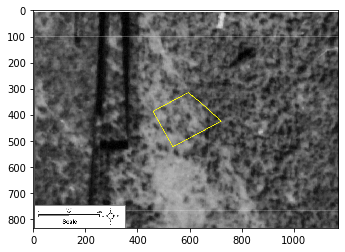Next, we'll create the mask image. We start with conversion to HSV - this is hue-saturation-value, which is another way to represent image data. We find the outline by checking for portions of the image whose hue is particularly high.

After we detect the outline, we can show it in our notebook as a "mask image."

In :
hsv_img = rgb2hsv(image)
hsv_img[:,:,2] = hsv_img[:,:,0] > 0.16
hsv_img[:,:,1] = hsv_img[:,:,0] > 0.16
hsv_img[:,:,0] = hsv_img[:,:,0] > 0.16

Out:
<matplotlib.image.AxesImage at 0x7f60104aeda0>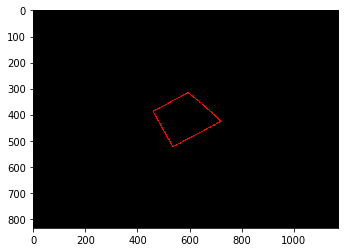## Getting Neighbors

We'll define a function for getting the neighbors of any particular pixel. We'll use this function later.

In :
def getNeighbours(i, j, n, m) :
arr = []
if i-1 >= 0 and j-1 >= 0 :
arr.append((i-1, j-1))
if i-1 >= 0 :
arr.append((i-1, j))
if i-1 >= 0 and j+1 < m :
arr.append((i-1, j+1))
if j+1 < m :
arr.append((i, j+1))
if i+1 < n and j+1 < m :
arr.append((i+1, j+1))
if i+1 < n :
arr.append((i+1, j))
if i+1 < n and j-1 >= 0 :
arr.append((i+1, j-1))
if j-1 >= 0 :
arr.append((i, j-1))
return arr


## Find the Inside and Outside

In this cell, we find the inside and the outside of the outlined section, and color the outside. We do this by starting at the corner of the image, and coloring the neighbors of each given pixel.

In :
n = image.shape
m = image.shape
queue = [(0, 0)]
while len(queue) > 0:
p = queue.pop()
if mask_image[p, p, 0] == 0 :
arr = getNeighbours(p, p, n, m)
for a in arr:
queue.append(a)


Out:
<matplotlib.image.AxesImage at 0x7f5ff63f3208>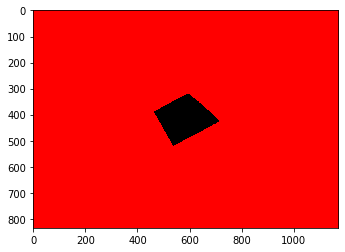## Saving the Outlined Section

We'll find the left, right, top, and bottom of the outlined section, by finding which parts of the mask are colored and uncolored.

In :
left = image.shape
right = 0
top = image.shape
bottom = 0

for i in range(n):
for j in range(m):
if mask_image[i, j, 0] != 1.0:
if i < top: top = i
if i > bottom: bottom = i
if j < left: left = j
if j > right: right = j

alpha_image = alpha_image[top:bottom+1, left:right+1, :]
plt.imshow(alpha_image)

Out:
<matplotlib.image.AxesImage at 0x7f60103ea400>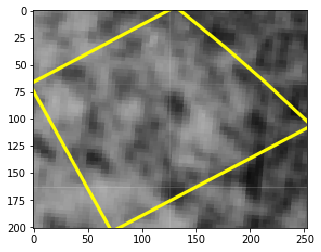## Removing the Outside Sections

We'll continue our isolation of the outlined section in this cell.

In :
pixel_count = 0

for i in range(alpha_image.shape):
for j in range(alpha_image.shape):
if mask_image[i, j, 0] == 1.0:
alpha_image[i, j, 3] = 0.0
else:
pixel_count += 1
plt.imshow(alpha_image)

Out:
<matplotlib.image.AxesImage at 0x7f6010447cf8>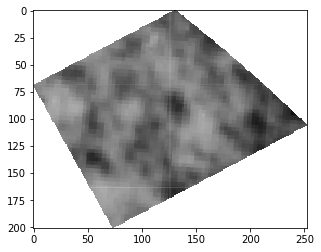## Saving the Results

Finally, we can save the results, and also check how big the outlined section is compared to the full image.

In :
io.imsave("result.png", alpha_image)
original_size = image.shape * image.shape
print ("Image size: {} pixels".format(pixel_count))
if False:
print(pixel_count)
print(original_size)
n = image.shape
m = image.shape
print('mandn')
print(n)
print(m)
print('topandbottom')
print(top)
print(bottom)
print(left)
print(right)
print ("Image promience: {0:.2f}% of original document image".format(pixel_count / original_size * 100))

Image size: 26863 pixels
Image promience: 2.75% of original document image

In [ ]: